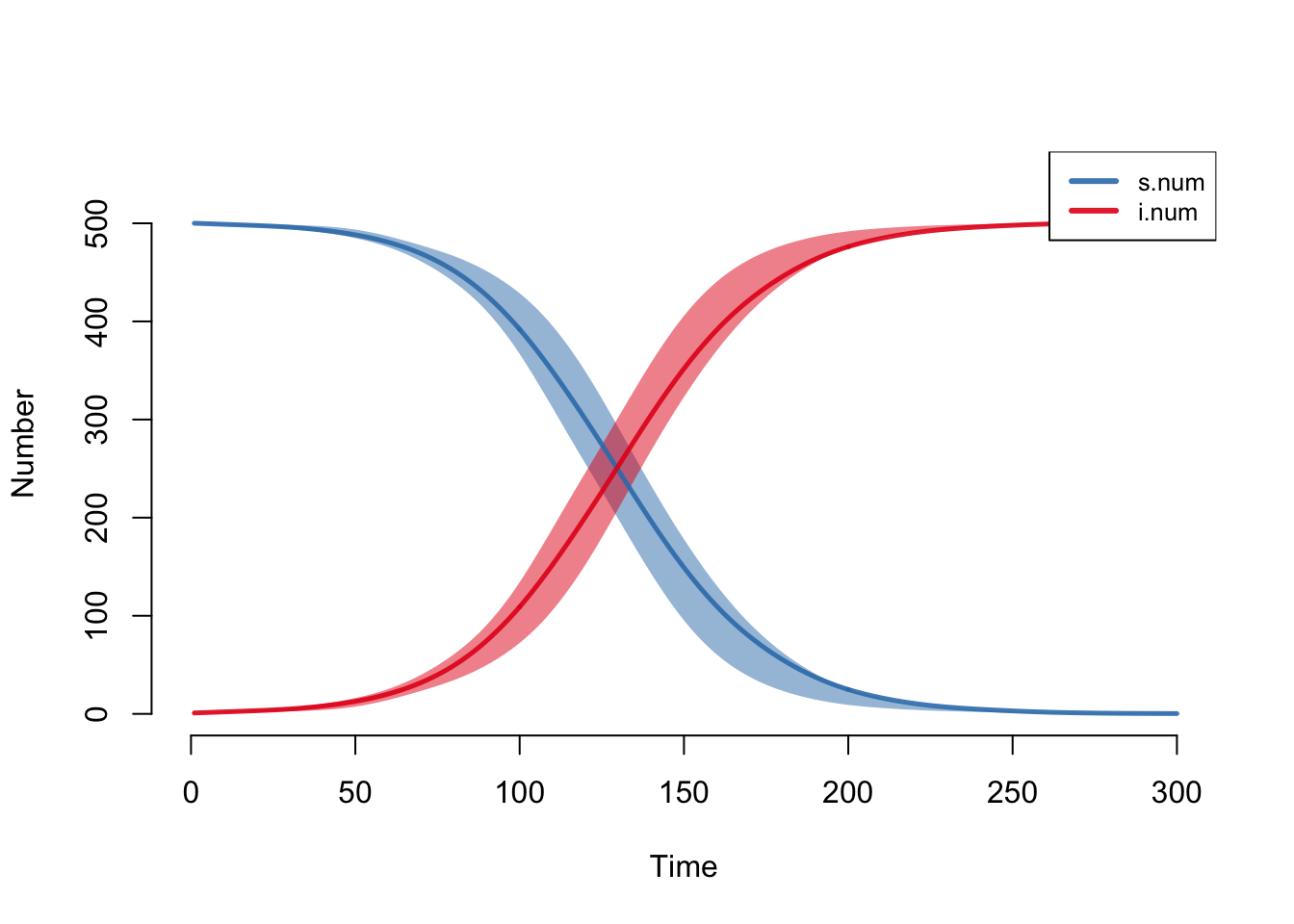# Introduction

This tutorial provides a mathematical and theoretical background for stochastic individual contact models (ICMs), with instructions on how to simulate the built-in models designed for learning stochastic modeling in EpiModel. If you are new to epidemic modeling, we suggest that you start with our tutorial Basic DCMs with EpiModel to get a background in that standard epidemic modeling approach. Some of the material here assumes familiarity with DCMs.

Stochastic ICMs are designed to be agent-based microsimulation analogs to the deterministic compartmental models (DCMs) solved with the `dcm` function. The main differences between the two model classes are as follows.

1. Parameters are random draws: these are stochastic models in which all of the rates and risks governing transitions between states are random draws from distributions summarized by those rates or probabilities, including normal, Poisson, and binomial distributions.
2. Time is discrete: ICMs are simulated in discrete time, in contrast to the continuous time of DCMs. In discrete time, everything within a time step happens as a series of processes, since there is no instantaneous occurrence of events independent of others, possible in continuous-time models. This has the potential to introduce some artificiality into the modeling if the unit for the time step is large because transitions that might occur within that time step cannot necessarily be considered independent. In general, caution is needed when simulating any discrete-time model with long unit time steps or large rate parameters, given the potential for competing risks.
3. Units are individuals: ICMs simulate disease spread over a simulated population of individually identifiable elements; in contrast, DCMs treat the population as an aggregate whole which is infinitely divisible, with individual elements in this population neither identifiable nor accessible for modeling purposes. The implications of this are relatively minor if the stochastic simulations occur on a sufficiently large population, but there are some critical modeling considerations of individual-based simulations that will be reviewed in the examples below.

A goal of EpiModel is to facilitate comparisons across different model classes, so the interface and functionality of the stochastic models in `icm`, the main function to run ICMs, is very similar to `dcm`, the main function to run DCMs. The syntax and argument names for the parameters and initial state sizes are the same.

# SI Model Stochasticity

We introduce ICM class models by using the same model parameterization as the basic DCM SI model the Basic DCMs Tutorial, with one person infected, an act rate of 0.25 per time step, and an infection probability of 0.2 per act. We simulate this model 10 times over 300 time steps. Note that the parameterization helper functions now end with `.icm`.

``````param <- param.icm(inf.prob = 0.2, act.rate = 0.25)
init <- init.icm(s.num = 500, i.num = 1)
control <- control.icm(type = "SI", nsims = 10, nsteps = 300)
mod <- icm(param, init, control)``````

By default, the function prints the model progress. These agent-based simulation models generally take longer to run than DCMs, especially for larger populations and longer time ranges, because transition processes must be simulated for each individual at each time step. The model results may be printed, summarized, and plotted in very similar a fashion to the `dcm` models.

``mod``
``````EpiModel Simulation
=======================
Model class: icm

Simulation Summary
-----------------------
Model type: SI
No. simulations: 10
No. time steps: 300
No. groups: 1

Model Parameters
-----------------------
inf.prob = 0.2
act.rate = 0.25

Model Output
-----------------------
Variables: s.num i.num num si.flow``````

In contrast to `dcm` class objects, the summary function does not take a `run` argument. The output summarizes the mean and standard deviation of model results at the requested time step across all simulations. Here, we request those summaries at time step 125; the output shows the compartment and flow averages across those 10 simulations.

``summary(mod, at = 125)``
``````EpiModel Summary
=======================
Model class: icm

Simulation Details
-----------------------
Model type: SI
No. simulations: 10
No. time steps: 300
No. groups: 1

Model Statistics
------------------------------
Time: 125
------------------------------
mean      sd    pct
Suscept.  276.1  93.136  0.551
Infect.   224.9  93.136  0.449
Total     501.0   0.000  1.000
S -> I      5.2   1.874     NA
------------------------------ ``````

Summary statistic values like means and standard deviations may be of interest for analysis and visualization, so the `as.data.frame` function for `icm` objects provides this. As described in the function help page (see `help("as.data.frame.icm")`), the output choices are for the time-specific means, standard deviations, and individual simulation values.

``head(as.data.frame(mod, out = "mean"))``
``````  time s.num i.num num si.flow
1    1 500.0   1.0 501     0.0
2    2 500.0   1.0 501     0.0
3    3 499.8   1.2 501     0.2
4    4 499.7   1.3 501     0.1
5    5 499.6   1.4 501     0.1
6    6 499.5   1.5 501     0.1``````
``tail(as.data.frame(mod, out = "vals", sim = 1))``
``````    sim time s.num i.num num si.flow
295   1  295     0   501 501       0
296   1  296     0   501 501       0
297   1  297     0   501 501       0
298   1  298     0   501 501       0
299   1  299     0   501 501       0
300   1  300     0   501 501       0``````

## Plotting

Plotting stochastic model results requires thinking through what summary measures best represent the epidemic. For some models, it may be sufficient to plot the simulation means, but in others visualizing the individual simulations is necessary. The plotting function for `icm` objects generates these visual outputs simply but robustly. Standard plotting output includes the means across simulations along with the inter-quartile range of values; both are smoothed values by default.

``plot(mod)``Each of the elements may be toggled on or off using the plotting arguments listed in `help("plot.icm")`. Here, we add the individual simulation lines to the default plot using `sim.lines` and remove the smoothing of the means and quantile band.

``plot(mod, y = "i.num", sim.lines = TRUE, mean.smooth = FALSE, qnts.smooth = FALSE)``